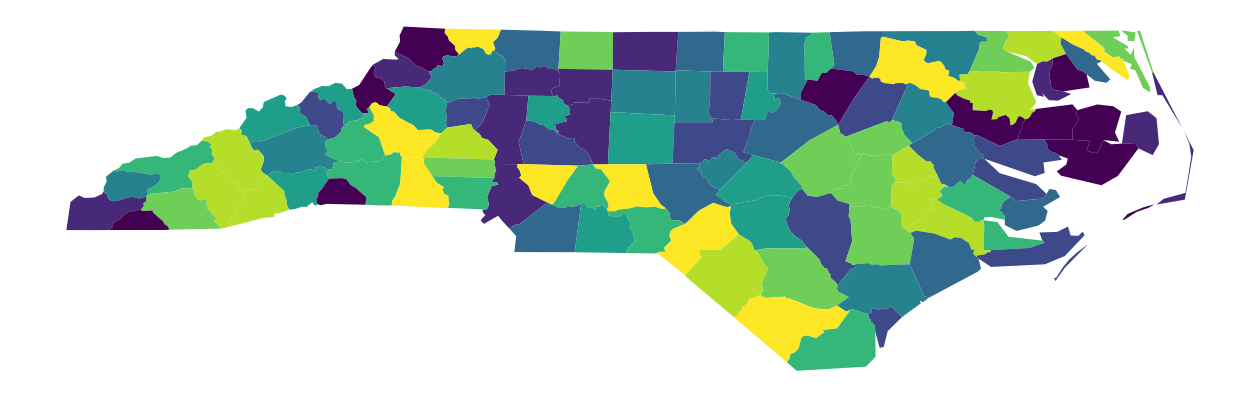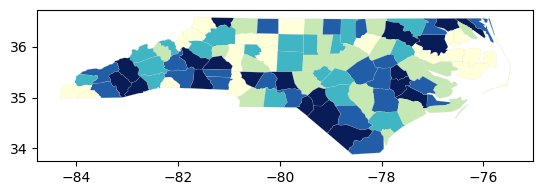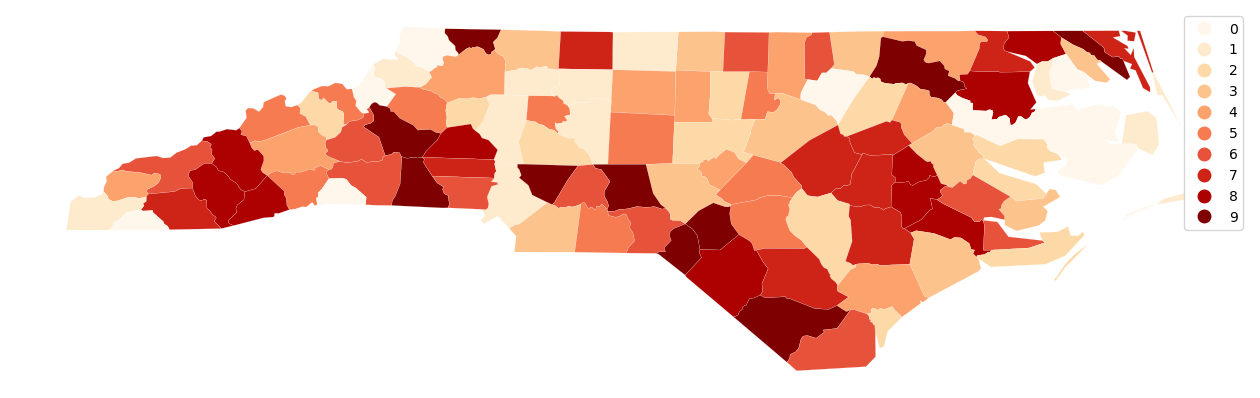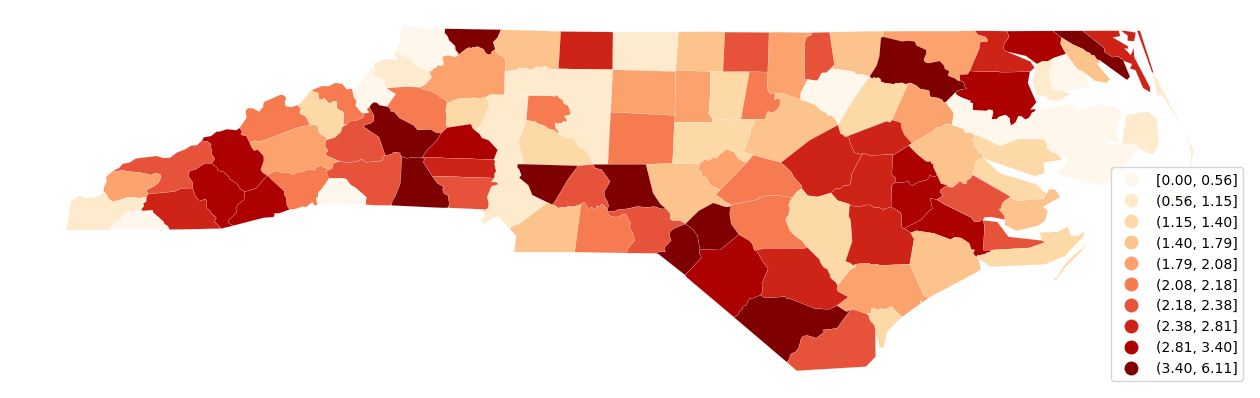# Choropleth¶

mapclassify is intended to be used with visualizaiton packages to handle the actual rendering of the choropleth maps defined on its classifiers. In this notebook, we explore some examples of how this is done. The notebook also includes an example that combines mapclassify with ipywidgets to allow for the interactive exploration of the choice of:

• classification method

• number of classes

• colormap

:

import libpysal
import geopandas
import mapclassify
import matplotlib.pyplot as plt

mapclassify.__version__

:

'2.4.2+78.gc62d2d7.dirty'


The example in this notebook uses data on sudden death infant syndrome for counties in North Carolina. It is a built-in dataset available through libpysal. We use libpysal to obtain the path to the shapefile and then use geopandas to create a geodataframe from the shapefile:

:

libpysal.examples.explain("sids2")

sids2
=====

North Carolina county SIDS death counts and rates
-------------------------------------------------

* sids2.dbf: attribute data. (k=18)
* sids2.shp: Polygon shapefile. (n=100)
* sids2.shx: spatial index.
* sids2.gal: spatial weights in GAL format.

Source: Cressie, Noel (1993). Statistics for Spatial Data. New York, Wiley, pp. 386-389. Rates computed.
Updated URL: https://geodacenter.github.io/data-and-lab/sids2/


:

pth = libpysal.examples.get_path("sids2.shp")

:

AREA PERIMETER CNTY_ CNTY_ID NAME FIPS FIPSNO CRESS_ID BIR74 SID74 NWBIR74 BIR79 SID79 NWBIR79 SIDR74 SIDR79 NWR74 NWR79 geometry
0 0.114 1.442 1825 1825 Ashe 37009 37009 5 1091.0 1.0 10.0 1364.0 0.0 19.0 0.916590 0.000000 9.165903 13.929619 POLYGON ((-81.47276 36.23436, -81.54084 36.272...
1 0.061 1.231 1827 1827 Alleghany 37005 37005 3 487.0 0.0 10.0 542.0 3.0 12.0 0.000000 5.535055 20.533881 22.140221 POLYGON ((-81.23989 36.36536, -81.24069 36.379...
2 0.143 1.630 1828 1828 Surry 37171 37171 86 3188.0 5.0 208.0 3616.0 6.0 260.0 1.568381 1.659292 65.244668 71.902655 POLYGON ((-80.45634 36.24256, -80.47639 36.254...
3 0.070 2.968 1831 1831 Currituck 37053 37053 27 508.0 1.0 123.0 830.0 2.0 145.0 1.968504 2.409639 242.125984 174.698795 MULTIPOLYGON (((-76.00897 36.31960, -76.01735 ...
4 0.153 2.206 1832 1832 Northampton 37131 37131 66 1421.0 9.0 1066.0 1606.0 3.0 1197.0 6.333568 1.867995 750.175932 745.330012 POLYGON ((-77.21767 36.24098, -77.23461 36.214...

Once created, the geodataframe has a plot method that can be called to create our first choropleth map. We will specify the column to classify and plot as SIDR79: SIDS death rate per 1,000 births (1979-84). The classification scheme is set to Quantiles, and the number of classes set to k=10 (declies):

:

ax = gdf.plot(column="SIDR79", scheme="Quantiles", k=10, figsize=(16, 9))
ax.set_axis_off()We can peak under the hood a bit and recreate the classification object that was used in the previous choropleth:

:

q10 = mapclassify.Quantiles(gdf.SIDR79, k=10)
q10

:

Quantiles

Interval     Count
--------------------
[0.00, 0.56] |    10
(0.56, 1.15] |    10
(1.15, 1.40] |    10
(1.40, 1.79] |    10
(1.79, 2.08] |    10
(2.08, 2.18] |    10
(2.18, 2.38] |    10
(2.38, 2.81] |    10
(2.81, 3.40] |    10
(3.40, 6.11] |    10


For quick, exploratory work, the classifier object has its own plot method that takes a geodataframe as an argument:

:

q10.plot(gdf);Back to working directly with the dataframe, we can toggle on the legend:

:

ax = gdf.assign(cl=q10.yb).plot(
figsize=(16, 9),
column="cl",
categorical=True,
k=10,
cmap="OrRd",
linewidth=0.1,
edgecolor="white",
legend=True,
)
ax.set_axis_off()Here we see the 10 classes, but without more specific information on the legend, the user has to know that 0 is the first decile and 9 the 10th. We also do not know the values that define these classes.

We can rectify this as follows:

:

q10.get_legend_classes()

:

['[0.00, 0.56]',
'(0.56, 1.15]',
'(1.15, 1.40]',
'(1.40, 1.79]',
'(1.79, 2.08]',
'(2.08, 2.18]',
'(2.18, 2.38]',
'(2.38, 2.81]',
'(2.81, 3.40]',
'(3.40, 6.11]']

:

mapping = dict([(i, s) for i, s in enumerate(q10.get_legend_classes())])
mapping

:

{0: '[0.00, 0.56]',
1: '(0.56, 1.15]',
2: '(1.15, 1.40]',
3: '(1.40, 1.79]',
4: '(1.79, 2.08]',
5: '(2.08, 2.18]',
6: '(2.18, 2.38]',
7: '(2.38, 2.81]',
8: '(2.81, 3.40]',
9: '(3.40, 6.11]'}

:

def replace_legend_items(legend, mapping):
for txt in legend.texts:
for k, v in mapping.items():
if txt.get_text() == str(k):
txt.set_text(v)

:

ax = gdf.assign(cl=q10.yb).plot(
figsize=(16, 9),
column="cl",
categorical=True,
k=10,
cmap="OrRd",
linewidth=0.1,
edgecolor="white",
legend=True,
legend_kwds={"loc": "lower right"},
)
ax.set_axis_off()
replace_legend_items(ax.get_legend(), mapping)## Interactive Exploration of Choropleth Classification¶

Next, we develop a small application that relies on mapclassify together with palettable and ipywidgets to explore the choice of:

• classification method

• number of classes

• colormap

:

from ipywidgets import (
interact,
Button,
Dropdown,
FloatSlider,
HBox,
IntSlider,
Label,
Output,
Tab,
VBox,
)

from palettable import colorbrewer


Declare that our application shall have 3 options for color scheme…

:

data_type = RadioButtons(options=["Sequential", "Diverging", "Qualitative"])


… and define thise 3 colormaps based on colorbrewer.

:

sequential = colorbrewer.COLOR_MAPS["Sequential"]
diverging = colorbrewer.COLOR_MAPS["Diverging"]
qualitative = colorbrewer.COLOR_MAPS["Qualitative"]


Next, create value and name options to bind the radio button to the dropdown menus…

:

bindings = {
"Sequential": range(3, 9 + 1),
"Diverging": range(3, 11 + 1),
"Qualitative": range(3, 12 + 1),
}
cmap_bindings = {
"Sequential": list(sequential.keys()),
"Diverging": list(diverging.keys()),
"Qualitative": list(qualitative.keys()),
}
class_val = Dropdown(options=bindings[data_type.value], value=5)
cmap_val = Dropdown(options=cmap_bindings[data_type.value])


… and define 7 mapclassify objects data classification.

:

k_classifiers = {
"equal_interval": mapclassify.EqualInterval,
"fisher_jenks": mapclassify.FisherJenks,
"jenks_caspall": mapclassify.JenksCaspall,
"jenks_caspall_forced": mapclassify.JenksCaspallForced,
"maximum_breaks": mapclassify.MaximumBreaks,
"natural_breaks": mapclassify.NaturalBreaks,
"quantiles": mapclassify.Quantiles,
}


Now it all together with helper functions for visualizing & updating plot options.

:

def k_values(ctype, cmap):
k = list(colorbrewer.COLOR_MAPS[ctype][cmap].keys())
return list(map(int, k))

def update_map(method="quantiles", k=5, cmap="Blues"):
classifier = k_classifiers[method](gdf.SIDR79, k=k)
mapping = dict([(i, s) for i, s in enumerate(classifier.get_legend_classes())])
plt_kws = dict(figsize=(16, 9), column="cl", categorical=True, legend=True, lw=0.1)
ax = gdf.assign(cl=classifier.yb).plot(
k=k, cmap=cmap, ec="grey", legend_kwds={"loc": "lower left"}, **plt_kws
)
ax.set_axis_off()
ax.set_title("SIDR79")
replace_legend_items(ax.get_legend(), mapping)

def type_change(change):
class_val.options = bindings[change["new"]]
cmap_val.options = cmap_bindings[change["new"]]

def cmap_change(change):
cmap = change["new"]
ctype = data_type.value
k = k_values(ctype, cmap)
class_val.options = k


Finally, let’s play with the interactive plot! * the plot will not render in the docs, but may be accessed directly by running this notebook locally or as a binder.

:

data_type.observe(type_change, names=["value"])
cmap_val.observe(cmap_change, names=["value"])
out = Output()
Tab().children = [out]
interact(update_map, method=list(k_classifiers.keys()), cmap=cmap_val, k=class_val)
display(VBox([data_type, out]))


Changing the type of colormap (sequential, diverging, qualitative) will update the options for the available color maps (cmap). Changing any of the values using the dropdowns will update the classification and the resulting choropleth map.

It is important to note that the example variable is best portrayed with the sequential colormaps. The other two types of colormaps are included for demonstration purposes only.